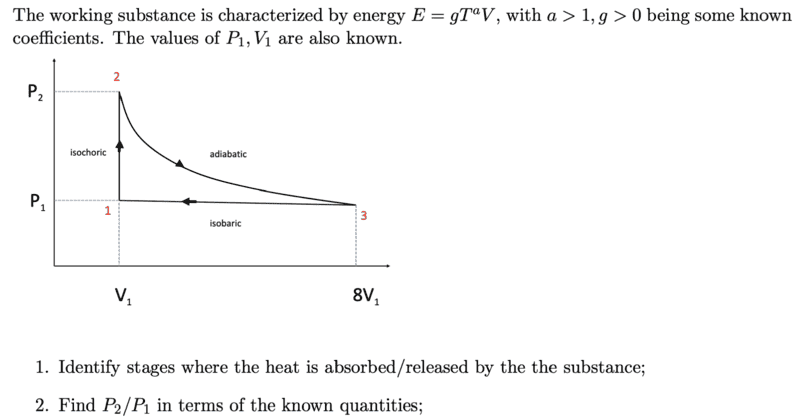# Calculating pressure from a known equation of energy

ForgetfulPhysicist
Homework Statement:
Heat Engine with working substance characterized by energy E = g T^a V, with a>1 and g>0 being known coeﬃcients. The values of P1, V1 are also known. Find P2 /P1 in terms of the known quantities.
Relevant Equations:
dE = TdS - PdVMy attempted solution is as follows:

Obviously the heat transfer happens during transitions 1->2 and 3->1.

It's also clear that
P1 = P3
V1 = V2

E2 - E1 = Integral[T dQ , from state 1 to state 2]

E3 - E2 = - Integral[P dV , from state 2 to state 3]

E1 - E3 = Integral[T dQ , from state 3 to state 1] + 7 P1 V1

But I can't find a way to perform any of these integrals or make any progress on this problem.

An attempt to calculate pressure is stuck at: p = - (dE/dV)_S = g a T^(a-1) (dT/dV)_S V + g T^a

Last edited:

Philip Koeck
E2 - E1 = Integral[T dQ , from state 1 to state 2]

E1 - E3 = Integral[T dQ , from state 3 to state 1] + 7 P1 V1
Check the units in these equations.

ForgetfulPhysicist
Check the units in these equations.
Yes that was a typo. They should be written:
E2 - E1 = Integral[dQ , from state 1 to state 2]
E1 - E3 = Integral[dQ , from state 3 to state 1] + 7 P1 V1

One idea: I can calculate Cv = (dE/dT)_V = a g T^(a-1) V , and I also know Cv = T (dS/dT)_V which helps me know a little bit about the change of entropy from state 1 to 2.... but I'm still stuck.

Last edited:
Mentor
Yes that was a typo. They should be written:
E2 - E1 = Integral[dQ , from state 1 to state 2]
E1 - E3 = Integral[dQ , from state 3 to state 1] + 7 P1 V1

One idea: I can calculate Cv = (dE/dT)_V = a g T^(a-1) V , and I also know Cv = T (dS/dT)_V which helps me know a little bit about the change of entropy from state 1 to 2.... but I'm still stuck.
I also made a little progress on this by using $$\left(\frac{\partial E}{\partial V}\right)_T=-\left[P-T\left(\frac{\partial P}{\partial T}\right)_V\right]=gT^a$$which leads to $$P=\frac{gT^a}{a-1}+Tf(V)$$

•Philip Koeck
Mentor
I derived the equation for entropy variation also, but I've as yet not been able to figure out a way that it can be used to provide an answer to this problem: $$dS=\frac{ga}{(a-1)}d(T^{a-1}V)+f(V)dV$$

•Philip Koeck
Mentor
From dimensional considerations, it makes sense to me that the function f(V) should be proportional to R/V, where R is the gas constant. If f(V) were equal to R/v, we would have $$\frac{PV}{RT}=z=1+\frac{1}{a-1}\frac{E}{RT}$$I'm unable to prove this yet, but I may proceed as if it is the case and see where it takes me.